Stochastic compartmental modelling with Stochastic Differential Equations

[In this module, students will become familiar with stochastic modelling with Stochastic Differential Equations for compartmental models and how they compare and contrast with Markov Chain Monte Carlo Methods.]

A good, but much more mathematical, introduction to the material discussed here can be found in the paper Construction of Equivalent Stochastic Differential Equation Models by Allen et al (2008).

Stochastic differential equations for compartmental models take the deterministic model, and add stochastic terms onto the model ODE’s to simulate random noise in the system.

An SDE model begins with a deterministic system of differential equations, describing the dynamics of a model with K compartments:where the f_1,… f_K are the functions of vec(X) for that model compartment.

If the model has J possible transitions of +/-1 individuals in the compartments, the SDE adds stochastic terms onto the deterministic model as follows:

where the terms of the form sum(G_kj W_j’) are the stochastic additions to the model, and involve a KxJ matrix, G, and J white noise terms, W’.  These  noise terms are independently sampled from the Normal distribution N(0,1), known as Wiener processes (we’ll refer to them as W from here on in).

In this past module, we discussed a formalism for preparing to perform compartmental modelling; a formalism that involves tabulating the possible state transitions of +/-1 individual into a matrix, lambda, and also tabulating the transition rates, p_j/delta_t.  It turns out that the G matrix is intimately related to lambda and the p vector, with G_kj = lambda_jk*sqrt(p_j/delta_t).

Example: SIR model

For instructive purposes, we’ll take the example of an SIR model. The deterministic model, with K=3 compartments, is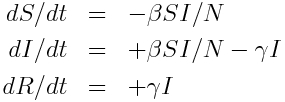The list of J=2 possible state transitions and rates is: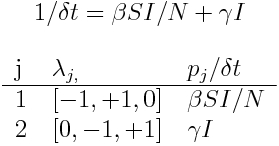Recall that the matrix lambda has J rows and K columns. To obtain the G matrix, first multiply each row of lambda by the square root of the transition rate for that row.  This yields:Now, simply take the transpose of that to obtain G, yielding: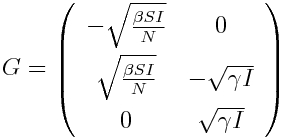This yields that the SDE for the SIR model is

Notice how I have written the equations to make it especially clear that if the transition is between two states, the sum of the relevant G matrix elements times the associated Wiener process has to sum to zero (ie; a fluctuation down in a compartment associated with a transition to another compartment has to be associated with a fluctuation up in the destination compartment).

What is the distribution of a Normally distributed random number X~N(0,1), multiplied by some number, A?  What are the mean and standard deviation of the Poisson distribution with parameter lambda? What distribution does the Poisson distribution approach in the limit of large lambda?
Based on the answers, what do the deterministic and stochastic terms represent in the above model?  And how do they relate to the SIR Poisson MCMC model, as seen in sir_markov_chain.R?

Notice that the deterministic components have units 1/time, but the stochastic components have units 1/sqrt(time).  This means that when using Euler’s method to numerically solve the system of equations, the change in each compartment is the deterministic part times delta_t, and the stochastic part time sqrt(delta_t).  The small changes in S, I, and R due to a small change in t are thus: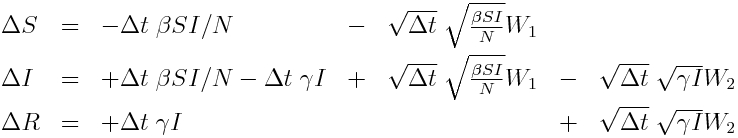The R script sir_sde_example.R shows how to numerically solve the system in Equation 1 using Euler’s method.  It produces the following plot: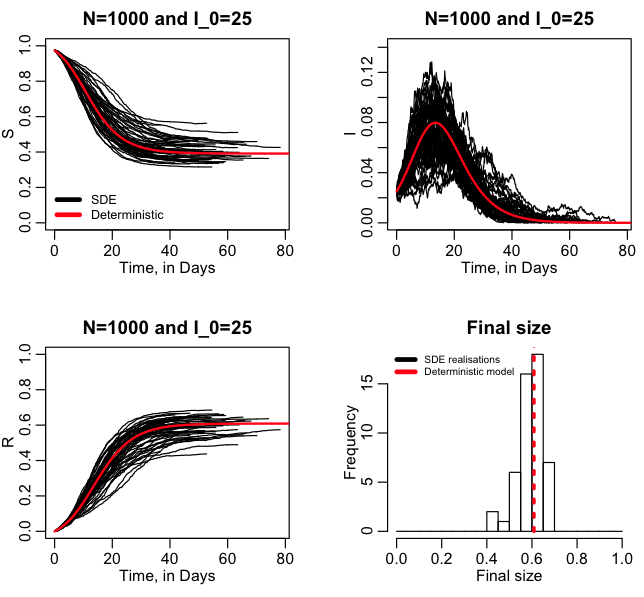Notice that I use a constant time step in the solution.  What would happen if I made this time step bigger?  or smaller?  Under what conditions could I have used a dynamically calculated time step?

In this past module, we compared and contrasted SDEs, MCMC, and agent based models.  When N=1000 and I_0=25 in an SIR model, is an SDE applicable?  How about an MCMC model?

The R script sir_sde_compare_mcmc.R compares the results of an SDE SIR model to a MCMC model.  It produces the following plot: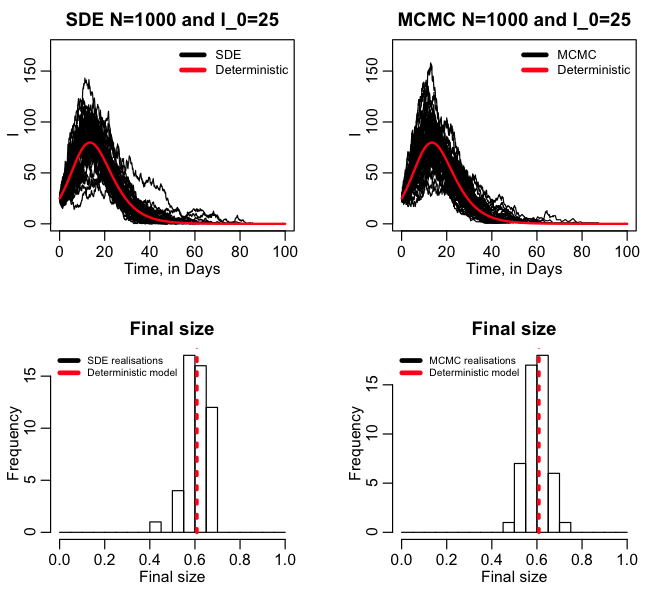At what points along the epidemic prevalence curve is the MCMC model more trustworthy than the SDE model?

Another example: SEIR model

The equations for the Susceptible, Exposed, Infected, Recovered model are: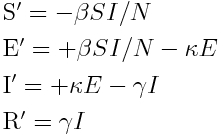Tabulating the state transitions of +/-1 individual in the system, and the rates for those transitions yields: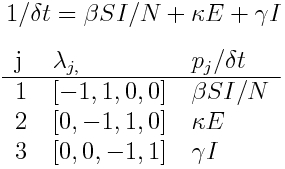Calculating the G matrix with G_kj = lambda_jk*sqrt(p_j/delta_t), yields: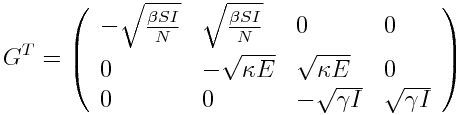Thus, we get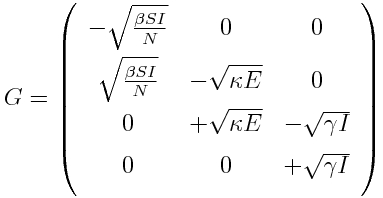The number of state transitions is J=3, thus we will be sampling three independent Wiener processes, to yield our SDE equations: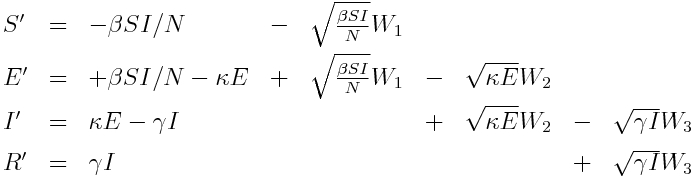The R script seir_sde_compare_mcmc.R performs 50 realisations of the above model, and compares it to the results of an MCMC model.  The script produces the plot: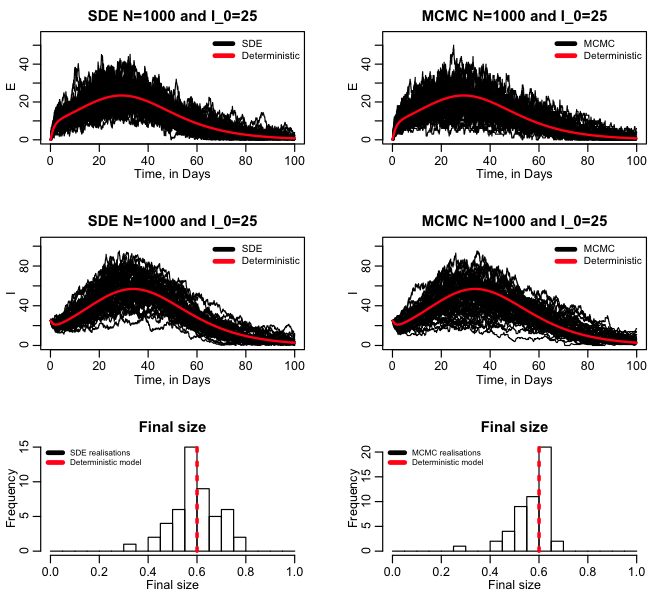Try plotting the results of the SDE model from the script (vS,vE,vI,vR) pairwise vs each other for the compartments. Then do the same, side by side, with the results from the MCMC model (wS, wE, wI, wR).  The covariance of the results from the two different methods should be similar.  In what domain are the results from the MCMC simulation?  How about the SDE simulation?

This is just one way to model SDE’s

The formalism I’ve presented above is just one way (and perhaps the simplest way for compartmental models) to set up stochastic models with SDE’s.  As is often the case, there is more than one way to skin the stochastic cat, and there are at least two other ways to set up a stochastic SDE, which are discussed in the paper Construction of Equivalent Stochastic Differential Equation Models by Allen et al (2008).

It is important to realise that if you are modelling a particular dynamical system, with a particular set of rules for mixing, probability distributions for sojourn time in compartments, etc, no matter what stochastic modelling method you are using, if you are using it appropriately with appropriate time steps, you have to get the same answer.  And by “same answer”, I mean the posterior probability distribution for your quantities of interest must be statistically indistinguishable between the methods.

Thus, if you code up your set of SDE equations for a model, run it for many realisations, and compare your results to that of an MCMC model (for instance), if the posterior probability distributions significantly differ, then at least one of the following must be true:

1. You are using an inappropriate time step in the SDE model
2. You are using the SDE model inappropriately if the number in any of the compartments is quite small; change the initial conditions to ensure that the compartments are populated enough, and rerun the SDE and MCMC simulations to compare.
3. You have a bug in your SDE code
4. You have a bug in your MCMC code